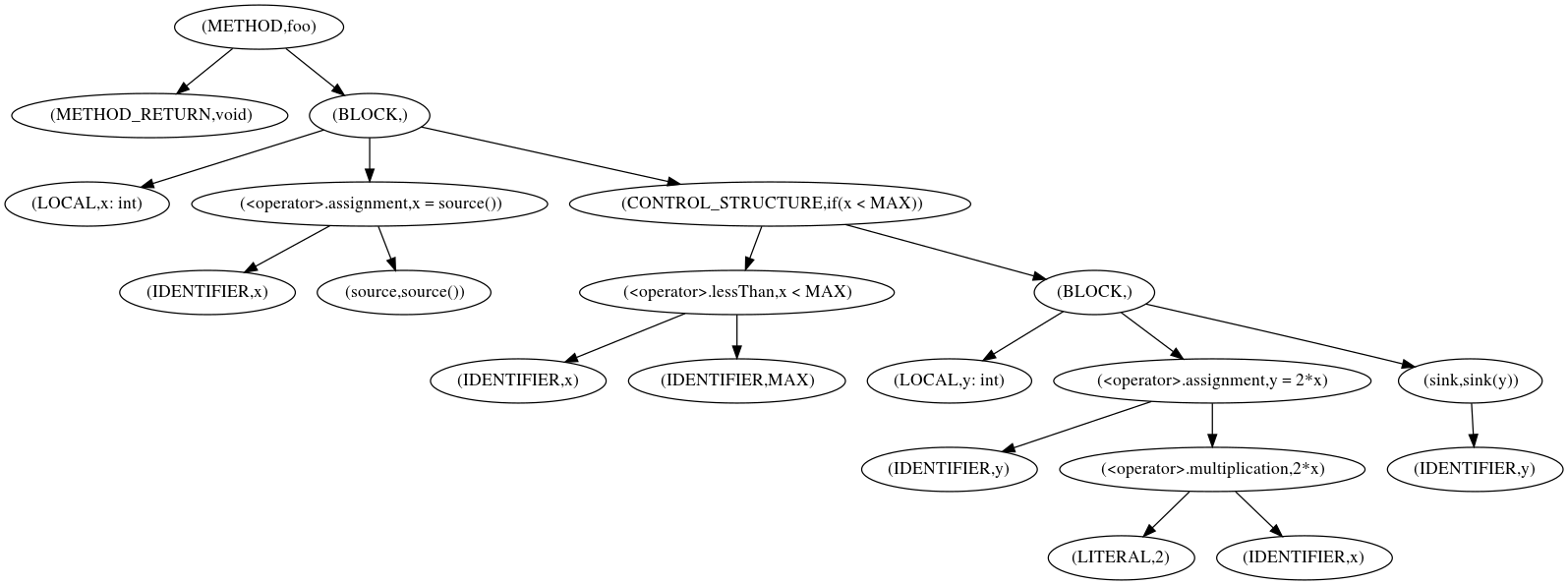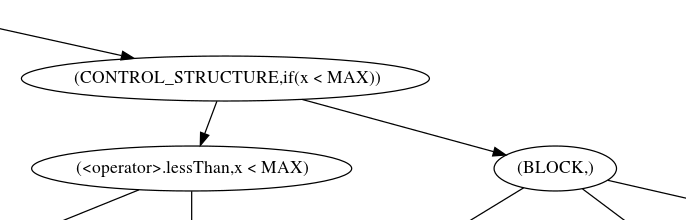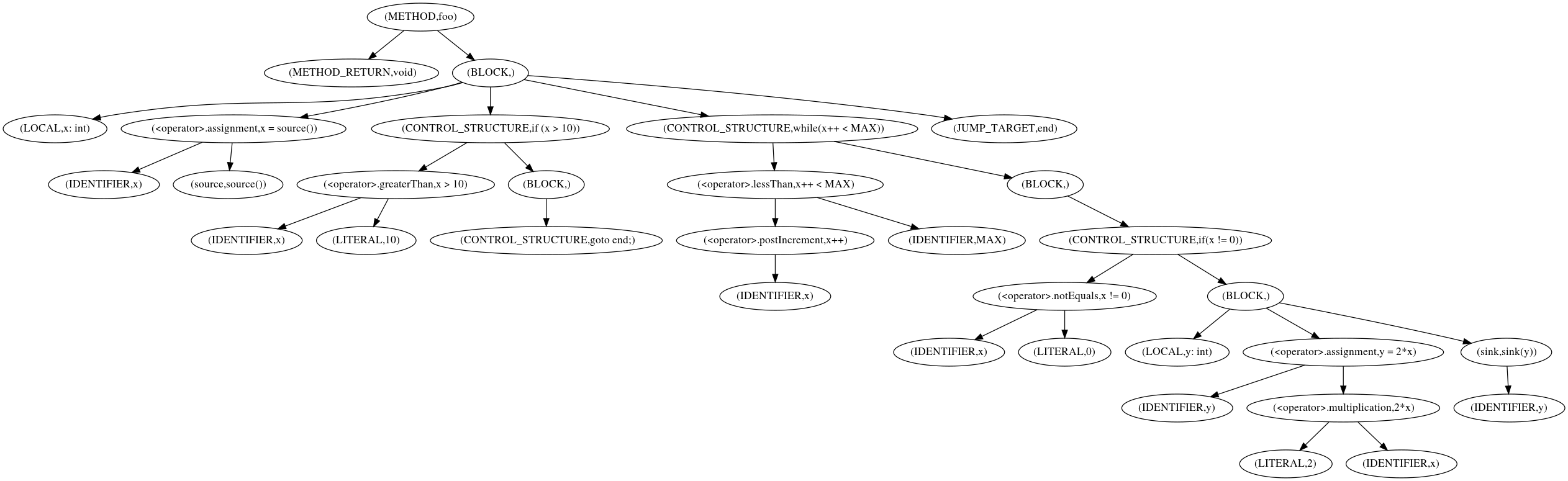# Syntax-Tree Queries

by Fabian Yamaguchi

In this article, we introduce readers to syntax-tree queries and show how they can be used as a computationally cheap approach for identifying code that handles attacker-controlled input or follows known bad practices. On the one hand, this article provides a practical introduction to mining code for patterns in its abstract syntax trees via a fluent query language. On the other hand, we discuss the limitations of syntax-tree queries at length.

## What are Abstract Syntax Trees?#

Throughout this article, we are using variations of the following small sample code snippet taken (taken from this paper):

val code = """
void foo () {
int x = source();
if(x < MAX) {
int y = 2*x;
sink(y);
}
}
"""

The snippet consists of a single function named `foo` without a return value. It calls a function called `source` on line 2 and stores its result in an integer called `x`. A subsequent check on line 3 determines whether `x` is smaller than a constant `MAX`, and if so, the value of `2*x` is stored in y on line 4 and passed as an argument to the function `sink` on line 5.

We can import this code snippet directly on the Ocular shell (see top level commands).

importCode.c.fromString(code)

Once imported, we can plot the abstract syntax tree of `foo` to get a first idea of what an abstract syntax tree is.

cpg.method.name("foo").plotDotAstFig.1: Abstract Syntax Tree for function `foo`. (Click to zoom in)

As shown in the sample plot in Figure 1, an abstract syntax tree is a tree-like structure that makes the decomposition of code into its language constructs explicit. The tree consists of nodes and edges visualized in the plot as ellipses and arrows respectively.

Nodes represent language constructs such as methods, variables, and control structures, while edges indicate decomposition. For example, at the very top of the tree, we see a node representing the entire function `foo`, and directly beneath it, we see a node for the return type `void` and one for the function body, denoted as a `BLOCK`. The edges between the function- and the two other nodes indicates that the function can be decomposed into a return type and a block of code, and outgoing edges from `BLOCK` show that this block of code can be further decomposed.

##### note

In the plot, the pair `(type,attribute)` is displayed in each node, where `type` is the node type and attribute is one of the values that is particularly indicative for the node, e.g., the method name for method nodes. We make an exception for calls, where we display the pair `(name,attribute)`.

## Basic AST Traversals#

The most basic traversal that you can execute on any AST node is `ast`, which traverses to all nodes of the AST rooted in the node. For example,

cpg.method("foo").ast.l

gives you all AST nodes of the AST for the method `foo`. As each AST node is also the root of a subtree, you can also think of this operation as an enumeration of all subtrees. These can be filtered by type. For example,

cpg.method("foo").ast.isCall.code.l

gives you all outgoing calls from `foo` while

cpg.method("foo").ast.isControlStructure.code.l

gives you all control structures. For method nodes, we also offer shorthands for the most common node types. Using these shorthands, the two queries can be written as

cpg.method("foo").call.code.l

and

cpg.method("foo).controlStructure.code.l

respectively.

It is also possible to walk the tree upwards using `inAst` or `inAstMinusLeaf` where the latter excludes the start node. In our running example, consider for example the situation where it is known that calls to `source` return values that an attacker can influence.

cpg.call.name("source").inAstMinusLeaf.isCall.name(".*assignment.*").argument(1).l

The query begins by selecting all calls to `source`, encoded in the graph as nodes of type `CALL` with a string property called `name` that is set to `"source"`. From there, we walk edges backwards until we reach the method node using `inAstMinusLeaf`. For the set of nodes thus collected, we determine only those which are calls (`isCall`) to the assignment operator `.name(".*assignment.*")`. For each of these, we determine their first argument, the assignment target.

As certain operators exist across programming languages and operating on them via AST-queries is common, we have created a decorator language to simplify these queries. In practice, we would write the following query that is equivalent to the former query.

cpg.call("source").inAssignment.target.l

## Control Structures#

Abstract syntax trees include control structures such as `if`-, `while` or `for`-statements. Our example program contains only a single control structure, namely, the `if`-statement on line 3.Fig.3: Control structure in the abstract syntax tree. The subtree on the left represents the condition, the subtree on the right is the compound statement.

Figure 3 shows how `if`-statements are represented in the syntax tree. The tree consists of two sub trees, one that holds the condition `x < MAX` and another that holds the statements in the `if`-block. Conditions can be selected as follows:

cpg.method("foo").controlStructure.condition.code.l
=> List("x < MAX")

The body of the if-statement can be selected using `whenTrue`:

cpg.method("foo").controlStructure.whenTrue.l
res16: List[AstNode] = List(
Block(
id -> 17L,
code -> "",
order -> 2,
argumentIndex -> 2,
typeFullName -> "void",
dynamicTypeHintFullName -> List(),
lineNumber -> Some(5),
columnNumber -> Some(4),
depthFirstOrder -> None,
internalFlags -> None
)
)

For `if-else` constructs, `whenFalse` returns the else block, however, since no `else` block exists in our example, an empty list is returned:

cpg.method("foo").controlStructure.whenFalse.l
=> List[AstNode] = List()

As each of the nodes returned by `.ast` are also roots of syntax trees, we can identify nested structures by chaining basic operations.

cpg.method("foo").controlStructure.whenTrue.ast.isCall.code.l
=> List[String] = List("y = 2*x", "sink(y)", "2*x")

yields all calls nested inside if blocks. You may ask yourself why `2*x` is returned as a call. The reason is that we model all invocations of built-in operators as calls to methods with the name `<operator>.\$operatorName`, where \$operatorName may for example be "multiplication", or "assignment".

One example scenario where control structure access comes in handy is when you wish to determine all methods that call a specific function but do not include a necessary check. Say, for example, that we want to identify functions that call `source` but they do not include a check against `MAX`. The following query achieves this:

cpg.method.filterNot(_.controlStructure(".*MAX.*"))
.filter(_.callee.name(".*source.*")).l
=> List()

Note, however, that the query does not specify the oder in which these statements must occur, e.g., the check may happen too late. Control flow graphs and dominator trees are the right tools to reason about statement order. These structures are available in the code property graph as well, but we do not discuss them in this article.

## Nesting of control structures#

Let us modify our example code slightly as follows to obtain a deeper understanding of what one can and cannot do using the control structures exposed by abstract syntax trees.

val code = """
void foo () {
int x = source();
if (x > 10) {
goto end;
}
while(x++ < MAX) {
if(x != 0) {
int y = 2*x;
sink(y);
}
}
end:
}
"""

Importing and plotting yields the following:Fig. 4: Abstract syntax tree containing structured and unstructured control structures

As we can see in Figure 4, the language formulated so far can be used easily to describe nested constructs. For example, if we would like to identify all calls to `sink` nested in an `if`-block that is itself nested in a `while` block, we could use the following query:

cpg.call("sink")
.inAst.filter(_.isControlStructure.code("if.*"))
.inAst.filter(_.isControlStructure.code("while.*")).l
=> List[AstNode] = List(
ControlStructure(
id -> 19L,
code -> "while(x++ < MAX)",
columnNumber -> Some(2),
lineNumber -> Some(9),
order -> 3,
parserTypeName -> "WhileStatement",
argumentIndex -> 3,
depthFirstOrder -> None,
internalFlags -> None
)
)

Alternatively, we can walk the tree from its top to achieve the same:

cpg.method
.controlStructure("while.*")
.ast.isControlStructure.code("if.*")
.ast.isCallTo("sink").l
=> List[Call] = List(
Call(
id -> 36L,
code -> "sink(y)",
name -> "sink",
order -> 2,
methodInstFullName -> None,
methodFullName -> "sink",
argumentIndex -> 2,
dispatchType -> "STATIC_DISPATCH",
signature -> "TODO assignment signature",
typeFullName -> "ANY",
dynamicTypeHintFullName -> List(),
lineNumber -> Some(12),
columnNumber -> Some(0),
resolved -> None,
depthFirstOrder -> None,
internalFlags -> None
)
)

While the syntax tree is not primarily concerned with exposing control flow, when a function only contains structured control structures such as `if` blocks or `while` loops, limited reasoning about control flow is possible. For example, since the call to `sink` is nested inside the `if` block and `while` loop, we can be certain that the conditions introduced by these two control structures are evaluated at least once before `sink` is called. We can also be certain that a sibling tree to the left is executed before a tree itself.

This, however, does not work in the face of unstructured control flow as introduced by `goto`. We make these statements as well as their jump targets visible in the tree, however, allowing functions where control flow must be analyzed with control flow graphs can be determined.